Download the eSaral app and start learning from Kota's top IITians and doctors.

# Chemical Bonding - JEE Main Previous Year Questions with Solutions

JEE Main Previous Year Papers Questions of Chemistry With Solutions are available at eSaral. Simulator   Previous Years AIEEE/JEE Mains Questions
Q. The bond dissociation energy of B–F in $\mathrm{BF}_{3}$ is 646 kJ $\mathrm{mol}^{-1}$whereas that of C–F in $\mathrm{CF}_{4}$ is 515 kJ mol–1. The correct reason for higher B–F bond dissociation energy as compared to that of C–F is :- (1) Significant $\mathrm{p} \pi-\mathrm{p} \pi$ interaction between B and F in $\mathrm{BF}_{3}$whereas there is not possibility of such interaction between C and F in $\mathrm{CF}_{4}$. (2) Lower degree of p – p interaction between B and F in $\mathrm{BF}_{3}$ than that between C and F in $\mathrm{CF}_{4}$ (3)Smaller size of B-atom as compared to that of C-atom (4) Stronger  bond between B and F in $\mathrm{BF}_{3}$ as compared to that between C and F in $\mathrm{CF}_{4}$ [AIEEE-2009]
Ans. (1) Due to back bonding in BF3 molecule all B–F bond having partial double bond character.
Q. Using MO theory predict which of the following species has the shortest bond length ? (1) $\mathrm{o}_{2}^{-}$ (2) $\mathrm{O}_{2}^{2-}$ (3) $\mathrm{O}_{2}^{2+}$ (4) $\mathrm{O}_{2}^{+}$ [AIEEE-2009]
Ans. (3)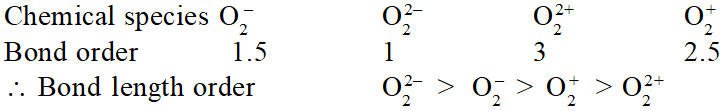Q. The hybridisation of orbitals of N atom in $\mathrm{NO}_{3}^{-}, \mathrm{NO}_{2}^{+}$ and $\mathrm{NH}_{4}^{+}$ are respectively:- [AIEEE-2011]
Ans. (4)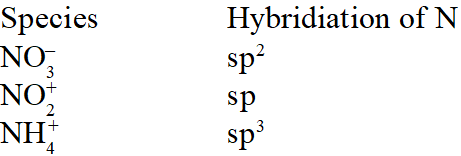Q. The structure of $\mathrm{IF}_{7}$ is :- (1) octahedral (2) pentagonal bipyramid (3) square pyramid (4) trigonal bipyramid [AIEEE-2011]
Ans. (2)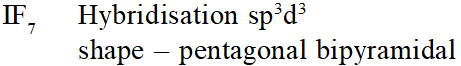Q. Among the following the maximum covalent character is shown by the compound :- (1) $\mathrm{AlCl}_{3}$ (2) $\mathrm{MgCl}_{2}$ (3) FeCl $_{2}$ (4) $\mathrm{SnCl}_{2}$ [AIEEE-2011]
Ans. (1) $\mathrm{Al}^{+3}$ having highest polarizing power than other : having greater covalent character
Q. Which of the following has maximum number of lone pairs associated with Xe (1) $\mathrm{XeO}_{3}$ (2) $\mathrm{XeF}_{4}$ (3) XeF $_{6}$ (4) $\mathrm{XeF}_{2}$ [AIEEE-2011]
Ans. (4)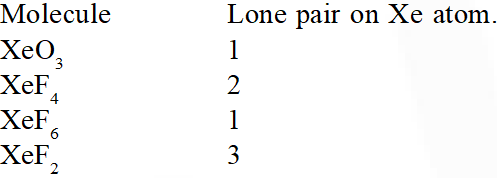Q. The number of types of bonds between two carbon atoms in calcium carbide is :- (1) One sigma, two pi (2) One sigma, one pi (3) Two sigma, one pi (4) Two sigma, two pi [AIEEE-2011]
Ans. (1) $\mathrm{Ca}^{+2}(\mathrm{C} \equiv \mathrm{C})^{2-}$
Q. The molecule having smallest bond angle is :- (1) $\mathrm{PCl}_{3}$ (2) $\mathrm{NCl}_{3}$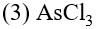(4) $\mathrm{SbCl}_{3}$ [AIEEE-2012]
Ans. (4) Bond angle order $\mathrm{NCl}_{3}>\mathrm{PCl}_{3}>\mathrm{AsCl}_{3}>\mathrm{SbCl}_{3}$
Q. In which of the following pairs the two species are not isostructural ? (1) $\mathrm{AIF}_{6}^{3-}$ and $\mathrm{SF}_{6}$ (3) $\mathrm{CO}_{3}^{2-}$ and $\mathrm{NO}_{3}^{-}$ (3) $\mathrm{PCl}_{4}^{+}$ and $\mathrm{SiCl}_{4}$ (4) $\mathrm{PF}_{5}$ and $\mathrm{BrF}_{5}$ [AIEEE-2012]
Ans. (4)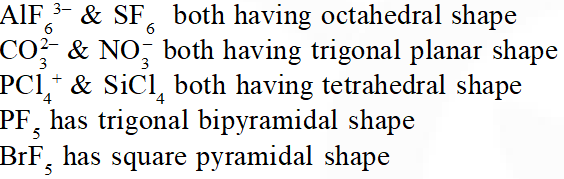Q. The number of S–S bonds in $\mathrm{SO}_{3}, \mathrm{S}_{2} \mathrm{O}_{3}^{2-}, \mathrm{S}_{2} \mathrm{O}_{6}^{2-}$ and $\mathrm{S}_{2} \mathrm{O}_{8}^{2-}$ respectively are :- (1) 1, 0, 1, 0 (2) 0, 1, 1, 0 (3) 1, 0, 0, 1 (4) 0, 1, 0, 1 [JEE-MAINS(Online) 2012]
Ans. (2)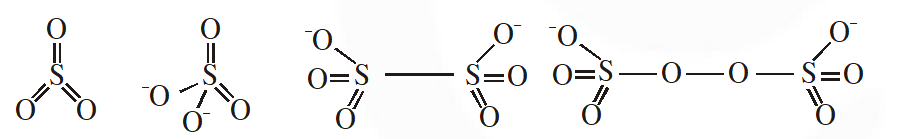Q. Dipole moment is shown by :- (1) trans-2, 3-dichloro- 2-butene (2) 1, 2-dichlorobenzene (3) 1, 4-dichlorobenzene (4) trans-1, 2-dinitroethene [JEE-MAINS(Online) 2012]
Ans. (2)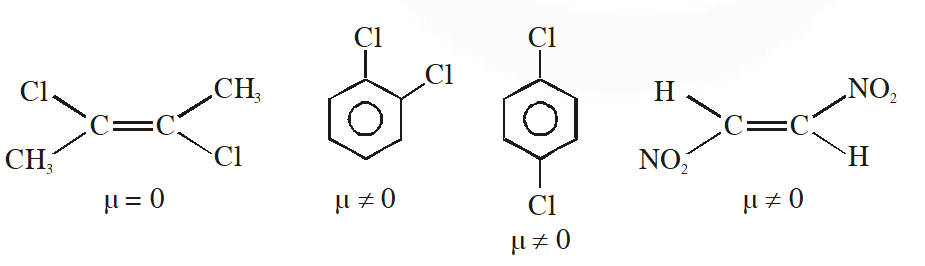Q. Among the following species which two have trigonal bipyramidal shape ?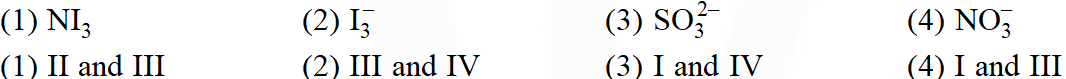[JEE-MAINS(Online) 2012]
Ans. (Bonus)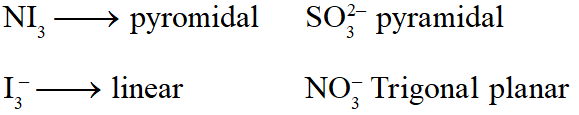Q. Among the following, the species having the smallest bond is :- (1) NO (2) $\mathrm{NO}^{+}$ (3) $\mathrm{O}_{2}$ (4) $\mathrm{NO}^{-}$ [JEE-MAINS(Online) 2012]
Ans. (2)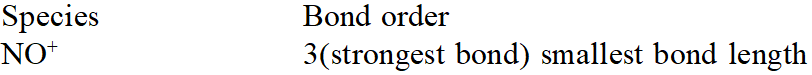Q. Based on lattice energy and other considerations, which one of the following alkali metal chloride is expected to have the highest melting point ? (1) RbCl (2) LiCl (3) KCl (4) NaCl [JEE-MAINS(Online) 2012]
Ans. (4) Highest melting is of NaCl
Q. Which of the following has the square planar structure :- (1) $\mathrm{NH}_{4}^{+}$ (2) $\mathrm{CCl}_{4}$ (3) $\mathrm{XeF}_{4}$ (4) $\mathrm{BF}_{4}^{-}$ [JEE-MAINS(Online) 2012]
Ans. (3)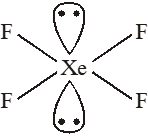Hybridisation $\mathrm{Sp}^{3} \mathrm{d}^{2}$ Shape – square planar
Q. The compound of Xenon with zero dipole moment is :- (1) $\mathrm{XeO}_{3}$ (2) $\mathrm{XeO}_{2}$ (3) $\mathrm{XeF}_{4}$ (4) $\mathrm{XeOF}_{4}$ [JEE-MAINS(Online) 2012]
Ans. (3)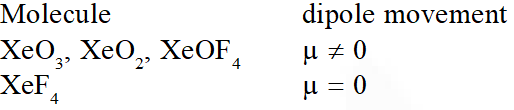Q. Among the following the molecule with the lowest dipole moment is :- (1) $\mathrm{CHCl}_{3}$ (2) $\mathrm{CH}_{2} \mathrm{Cl}_{2}$ (3) $\mathrm{CCl}_{4}$ (4) $\mathrm{CH}_{3} \mathrm{Cl}$ [JEE-MAINS(Online) 2012]
Ans. (3) $\mathrm{CCl}_{4}<\mathrm{CHCl}_{3}<\mathrm{CH}_{2} \mathrm{Cl}_{2}<\mathrm{CH}_{3} \mathrm{Cl}(\text { Dipolar moment })$
Q. The formation of molecular complex $\mathrm{BF}_{3}-\mathrm{NH}_{3}$ results in a change in hybridisation of boron (1) from $\operatorname{sp}^{3}$ to $\mathrm{sp}^{3} \mathrm{d}$ (2) from $\operatorname{sp}^{2}$ to $\mathrm{dsp}^{2}$ (3) from $\operatorname{sp}^{3}$ to $\mathrm{sp}^{2}$ (4) from $\operatorname{sp}^{2}$ to $\mathrm{sp}^{3}$ [JEE-MAINS(Online) 2012]
Ans. (4)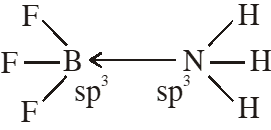Q. Among the following chloro-compound having the lowest dipole moment is :- (1) $\mathrm{CH}_{2} \mathrm{Cl}_{2}$ (2) $\mathrm{CH}_{3} \mathrm{Cl}$ (3)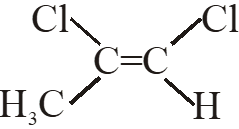(4)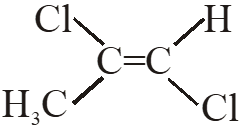Ans. (1) $\mathrm{CH}_{2} \mathrm{Cl}_{2}$ has lowest dipole moment
Q. Which one of the following molecules is expected to exhibit diamagnetic behaviour ? (1) $\mathrm{C}_{2}$ (2) $\mathrm{N}_{2}$ (3) $\mathrm{O}_{2}$ (4) $\mathrm{S}_{2}$ [AIEEE-2013]
Ans. (2) $\mathrm{N}_{2}$ is diamagnetic
Q. Which of the following is the wrong statement? (1) ONCl and $\mathrm{ONO}^{-}$ are not isoelectronic (2) $\mathrm{O}_{3}$ molecule is bent (3) Ozone is violet-black in solid state (4) Ozone is diamagnetic gas [JEE-maIN 2013]
Ans. (1) Number of electron in ONCl an $\mathrm{ONO}^{-}$ is 32 & 24 respectively.
Q. In which of the following pairs of molecules/ions, both the species are not likely to exist ? (1) $\mathrm{H}_{2}^{+}, \mathrm{He}_{2}^{2-}$ (2) $\mathrm{H}_{2}^{-}, \mathrm{He}_{2}^{2-}$ (3) $\mathrm{H}_{2}^{2+}, \mathrm{He}_{2}$ (4) $\mathrm{H}_{2}^{-}, \mathrm{H} \mathrm{e}_{2}^{2+}$ [JEE-main 2013]
Ans. (3) Bond order of $\mathrm{H}_{2}^{2+} \& \mathrm{He}_{2}$ is zero i.e. these molecule do not exist.
Q. Stability of the species $\mathrm{Li}_{2}, \quad \mathrm{Li}_{2}^{-}$ and $\mathrm{Li}_{2}^{+}$ increases in the order of :- (1) $\mathrm{Li}_{2}<\mathrm{Li}_{2}^{+}<\mathrm{Li}_{2}^{-}$ (2) $\mathrm{Li}_{2}^{-}<\mathrm{Li}_{2}^{+}<\mathrm{Li}_{2}$ (3) $\mathrm{Li}_{2}<\mathrm{Li}_{2}<\mathrm{Li}_{2}^{+}$ (4) $\mathrm{Li}_{2}^{-}<\mathrm{Li}_{2}<\mathrm{Li}_{2}^{+}$ [JEE-main 2013]
Ans. (2)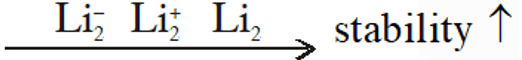Q. Trigonal bipyramidal geometry is shown by: (1) $\mathrm{XeO}_{3} \mathrm{F}_{2}$ (2) $\mathrm{XeOF}_{2}$ (3) $\left[\mathrm{XeF}_{8}\right]^{2-}$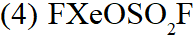[JEE-MAINS(Online) 2013]
Ans. (1)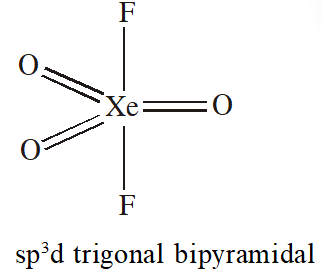Q. In which of the following ionization processes the bond energy has increased and also the magnetic behaviour has changed from paramagnetic to diamagnetic ? (1) $\mathrm{NO} \rightarrow \mathrm{NO}^{+}$ (2) $\mathrm{O}_{2} \rightarrow \mathrm{O}_{2}^{+}$ (3) $\mathrm{N}_{2} \rightarrow \mathrm{N}_{2}^{+}$ ( 4) $\mathrm{C}_{2} \rightarrow \mathrm{C}_{2}^{+}$ [JEE-MAINS(Online) 2013]
Ans. (1)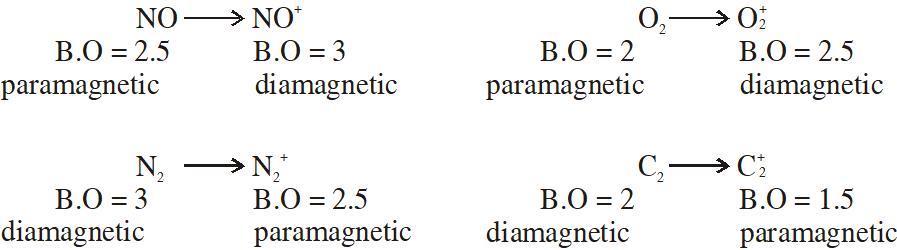Q. Which one of the following molecules is polar? (1) $\mathrm{CF}_{4}$ (2) $\mathrm{SbF}_{5}$ (3) $\mathrm{IF}_{5}$ (4) $\mathrm{XeF}_{4}$ [JEE-MAINS(Online) 2013]
Ans. (3)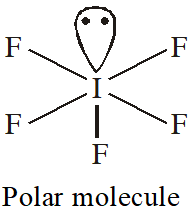Q. Oxidation state of sulphur in anions $\mathrm{SO}_{3,}^{2-} \mathrm{S}_{2} \mathrm{O}_{4}^{2-}$ and $\mathrm{S}_{2} \mathrm{O}_{6}^{2-}$ increases in the orders : (1) $\mathrm{S}_{2} \mathrm{O}_{6}^{2-}<\mathrm{S}_{2} \mathrm{O}_{4}^{2-}<\mathrm{SO}_{3}^{2-}$ (2) $\mathrm{SO}_{3}^{2-}<\mathrm{S}_{2} \mathrm{O}_{4}^{2-}<\mathrm{S}_{2} \mathrm{O}_{6}^{2-}$ (3) $\mathrm{S}_{2} \mathrm{O}_{4}^{2-}<\mathrm{SO}_{3}^{2-}<\mathrm{S}_{2} \mathrm{O}_{6}^{2-}$ (4) $\mathrm{S}_{2} \mathrm{O}_{4}^{2-}<\mathrm{S}_{2} \mathrm{O}_{6}^{2-}<\mathrm{SO}_{3}^{2-}$ [JEE-MAINS(Online) 2013]
Ans. (3)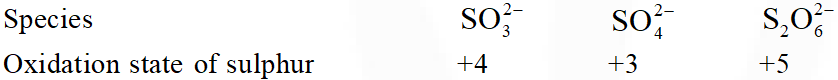Q. Bond order normally gives idea of stability of a molecular species. All the molecules viz. $\mathrm{H}_{2}, \mathrm{Li}_{2}$ and $\mathrm{B}_{2}$ have the same bond order yet they are not equally stable. Their stability order is: (1) $\mathrm{Li}_{2}>\mathrm{H}_{2}>\mathrm{B}_{2}$ (2) $\mathrm{H}_{2}>\mathrm{B}_{2}>\mathrm{Li}_{2}$ (3) $\mathrm{B}_{2}>\mathrm{H}_{2}>\mathrm{Li}_{2}$ (4) $\mathrm{Li}_{2}>\mathrm{B}_{2}>\mathrm{H}_{2}$ [JEE-MAINS(Online) 2013]
Ans. (2)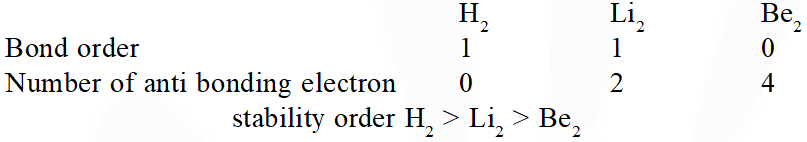Q. The solubility order for alkali metal fluoride in water is : (1) $\mathrm{LiF}<\mathrm{NaF}<\mathrm{KF}<\mathrm{RbF}$ (2) $\mathrm{LiF}>\mathrm{NaF}>\mathrm{KF}>\mathrm{RbF}$ (3) $\mathrm{RbF}<\mathrm{KF}<\mathrm{NaF}<\mathrm{LiF}$ (4) $\mathrm{LiF}<\mathrm{RbF}<\mathrm{KF}<\mathrm{NaF}$ [JEE-MAINS(Online) 2013]
Ans. (1) Solubility order LiF < NaF < KF < RbF
Q. $\mathrm{XeO}_{4}$ molecule is tetrahedral having : (1) Two p $\pi$ -d $\pi$ bonds (2) Four p $\pi$ - d $\pi$ bonds (3) One $\mathrm{p} \pi-\mathrm{d} \pi$ bond (4) Three p $\pi$ -d $\pi$ bonds [JEE-MAINS(Online) 2013]
Ans. (2)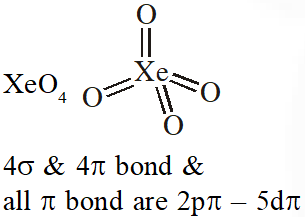Q. Bond distance in HF is 9.17 × $10^{-11}$ m. Dipole moment of HF is 6.104 × 10–30 Cm. The percent ionic character in HF will be : (electron charge = 1.60 × $10^{-19}$ C) (1) 61.0% (2) 38.0% (3) 35.5% (4) 41.5% [JEE-MAINS(Online) 2013]
Ans. (4)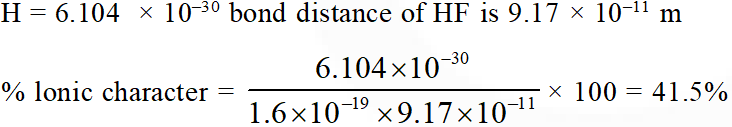Q. The shape of $\mathrm{IF}_{6}^{-}$ is : (1) Trigonally distorted octahedron (2) Pyramidal (3) Octahedral (4) Square antiprism [JEE-MAINS(Online) 2013]
Ans. (1)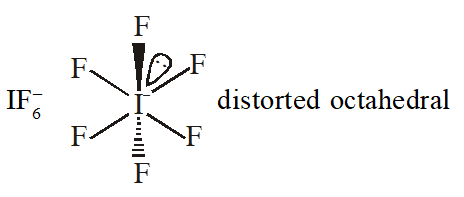Q. Which has trigonal bipyramidal shape ? (1) $\mathrm{XeOF}_{4}$ (2) $\mathrm{XeO}_{3}$ (3) $\mathrm{XeO}_{3} \mathrm{F}_{2}$ (4) $\mathrm{XeOF}_{2}$ [JEE-MAINS(Online) 2013]
Ans. (3)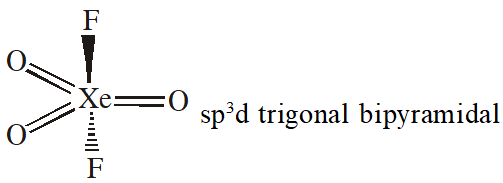Q. The catenation tendency of C, Si and Ge is in the order Ge < Si < C. The bond energies (in kJ $\mathrm{mol}^{-1}$) of C — C, Si —Si and Ge—Ge bonds are respectively : (1) 348, 260, 297 (2) 348, 297, 260 (3) 297, 348, 260 (4) 260, 297, 348 [JEE-MAINS(Online) 2013]
Ans. (2) Catenation/Bond energy order C – C > Si – Si > Ge – Ge
Q. In which of the following sets, all the given species are isostructural ? (1) $\mathrm{BF}_{3}, \mathrm{NF}_{3}, \mathrm{PF}_{3}, \mathrm{AlF}_{3}$ (2) $\mathrm{PCl}_{3}, \mathrm{AlCl}_{3}, \mathrm{BCl}_{3}, \mathrm{SbCl}_{3}$ (3) $\mathrm{BF}_{4}^{-}, \mathrm{CCl}_{4}, \mathrm{NH}_{4}^{+}, \mathrm{PCl}_{4}^{+}$ (4) $\mathrm{CO}_{2}, \mathrm{NO}_{2}, \mathrm{ClO}_{2}, \mathrm{SiO}_{2}$ [JEE-MAINS(Online) 2013]
Ans. (3) $\mathrm{BF}_{4}^{-}, \mathrm{CC}_{4}, \mathrm{NH}_{4}^{+}, \mathrm{PCl}_{4}^{+}$ are terahedral
Q. The internuclear distances in O —O bonds for O, $\mathrm{O}_{2}, \mathrm{O}^{-}$2 and O respectively are : (1) 1.49 Å, 1.21 Å, 1.12 Å, 1.30 Å (2) 1.30 Å, 1.49 Å, 1.12 Å, 1.21 (3) 1.12 Å, 1.21 Å, 1.30 Å, 1.49 Å (4) 1.21 Å, 1.12 Å, 1.49 Å, 1.30 Å [JEE-MAINS(Online) 2013]
Ans. (3)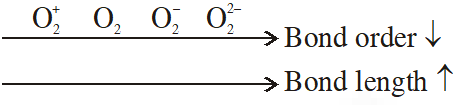Q. Which one of the following properties is not shown by NO ? (1) It combines with oxygen to form nitrogen dioxide (2) It's bond order is 2.5 (3) It is diamagnetic in gaseous state (4) It is a neutral oxide [JEE-main 2014]
Ans. (3)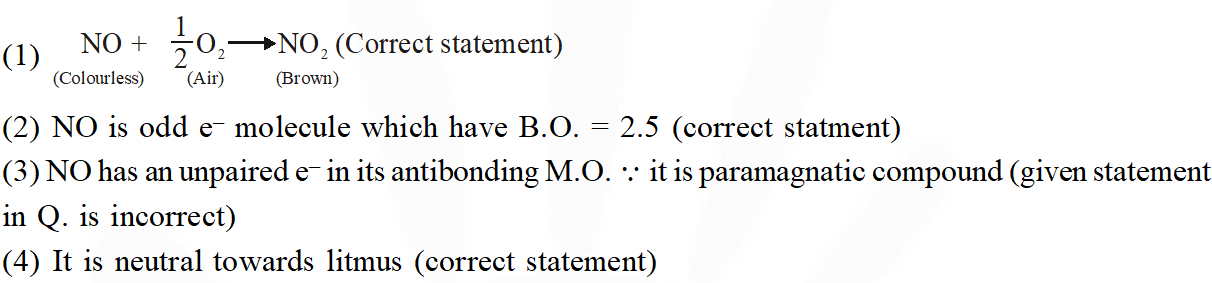Q. For which of the following molecule significant $\mu \neq 0$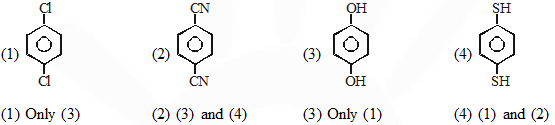[JEE-main 2014]
Ans. (2)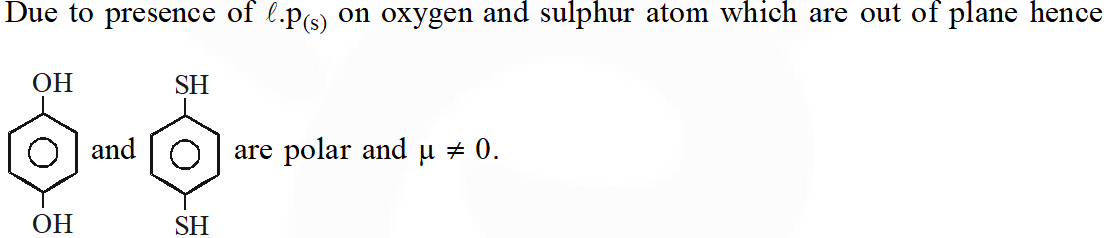Q. The number and type of bonds in $\mathrm{C}_{2}^{2-} \mathrm{ion}$ in $\mathrm{CaC}_{2}$ are: (1) Two $\sigma$ bonds and one $\pi-$ bond (2) Two $\sigma$ bonds and two $\pi-$ bonds (3) One $\sigma$ bond and two $\pi-$ bonds (4) One $\sigma$ bond and one $\pi-$ bond [JEE-MAINS(Online) 2014]
Ans. (3)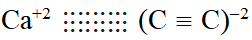Q. For the compounds $\mathrm{CH}_{3} \mathrm{Cl}, \mathrm{CH}_{3} \mathrm{Br}, \mathrm{CH}_{3} \mathrm{I}$ and $\mathrm{CH}_{3} \mathrm{F}$ (1) $\mathrm{CH}_{3} \mathrm{F}<\mathrm{CH}_{3} \mathrm{Br}<\mathrm{CH}_{3} \mathrm{Cl}<\mathrm{CH}_{3} \mathrm{I}$ (2) $\mathrm{CH}_{3} \mathrm{F}<\mathrm{CH}_{3} \mathrm{Cl}<\mathrm{CH}_{3} \mathrm{Br}<\mathrm{CH}_{3} \mathrm{I}$ (3) $\mathrm{CH}_{3} \mathrm{Cl}<\mathrm{CH}_{3} \mathrm{Br}<\mathrm{CH}_{3} \mathrm{F}<\mathrm{CH}_{3} \mathrm{I}$, the correct order of increasing C-halogen bond length is : (4) $\mathrm{CH}_{3} \mathrm{F}<\mathrm{CH}_{3} \mathrm{I}<\mathrm{CH}_{3} \mathrm{Br}<\mathrm{CH}_{3} \mathrm{Cl}$ [JEE-MAINS(Online) 2014]
Ans. (2)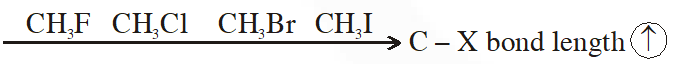Q. Which of the following has unpaired electron(s) ? (1) $\mathrm{O}_{2}^{-}$ (2) $\mathrm{N}_{2}^{2+}$ (3) $\mathrm{O}_{2}^{2-}$ (4) $\mathrm{N}_{2}$ [JEE-MAINS(Online) 2014]
Ans. (1) $\mathrm{O}_{2}^{-}$ ha one unpaired electron
Q. In allene $\left(\mathrm{C}_{3} \mathrm{H}_{4}\right)$, the type(s) of hybridization of the carbon atoms is (are): (1) only sp $^{2}$ (2) $\mathrm{sp}^{2}$ and $\mathrm{sp}$ (3) sp and sp $^{3}$ (4) $\mathrm{sp}^{2}$ and $\mathrm{sp}^{3}$ [JEE-MAINS(Online) 2014]
Ans. (2)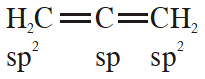Q. Shapes of certain interhalogen compounds are stated below. Which one of them is not correctly stated? (1) IF $_{7}:$ Pentagonal bipyramid (2) BrF $_{5}:$ Trigonal bipyramid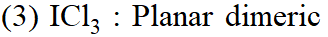(4) $\mathrm{BrF}_{3}:$ Planar T-shaped [JEE-MAINS(Online) 2014]
Ans. (2) $\mathrm{BrF}_{5}$ have square pyramidal shape.
Q. The correct order of bond dissociation energy among $\mathrm{N}_{2}, \mathrm{O}_{2}, \mathrm{o}_{2}^{-}$ is shown in which of the following arrangements? (1) $\mathrm{N}_{2}>\mathrm{O}_{2}>\mathrm{O}_{2}^{-}$ (2) $\mathrm{O}_{2}>\mathrm{O}_{2}^{-}>\mathrm{N}_{2}$ (3) $\mathrm{N}_{2}>\mathrm{O}_{2}^{-}>\mathrm{O}_{2}$ (4) $\mathrm{O}_{2}^{-}>\mathrm{O}_{2}>\mathrm{N}_{2}$ [JEE-MAINS(Online) 2014]
Ans. (1)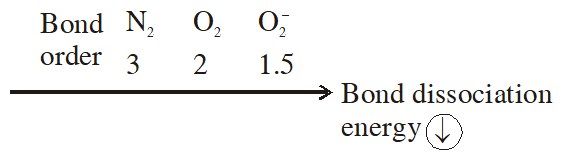Q. Which of the following molecules has two sigma$(\sigma)$ and two $\operatorname{pi}(\pi)$ bonds :- (1) HCN (2) $\mathrm{C}_{2} \mathrm{H}_{2} \mathrm{Cl}_{2}$ (3) $\mathrm{N}_{2} \mathrm{F}_{2}$ (4) $\mathrm{C}_{2} \mathrm{H}_{4}$ [JEE-MAINS(Online) 2014]
Ans. (1)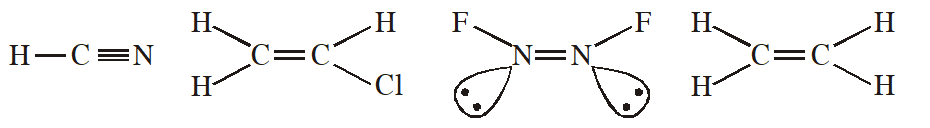Q. Which one of the following molecules is paramagnetic? (1) NO ( 2) $\mathrm{O}_{3}$ (3) $\mathrm{N}_{2}$ (4) CO [JEE-MAINS(Online) 2014]
Ans. (1) NO has unpaired e $^{-} \therefore$ paramagnetic is nature
Q. Amongst LiCl, RbCl, $\mathrm{BeCl}_{2}$ and $\mathrm{MgCl}_{2}$ the compounds with the greatest and the least ionic character, respectively are : (1) $\mathrm{RbCl}$ and $\mathrm{MgCl}_{2}$ (2) LiCl and RbCl (3) $\mathrm{MgCl}_{2}$ and $\mathrm{BeCl}_{2}$ (4) $\mathrm{RbCl}$ and $\mathrm{BeCl}_{2}$ [JEE-MAINS(Online) 2014]
Ans. (4) RbCl has highest ionic & and $\mathrm{BeCl}_{2}$ is most covalent
Q. The species in which the N atom is in a state of sp hybridization is :- (1) $\mathrm{NO}_{2}$ (2) $\mathrm{NO}_{2}^{+}$ (3) $\mathrm{NO}_{2}^{-}$ (4) $\mathrm{NO}_{3}^{-}$ [JEE-MAINS 2016]
Ans. (2)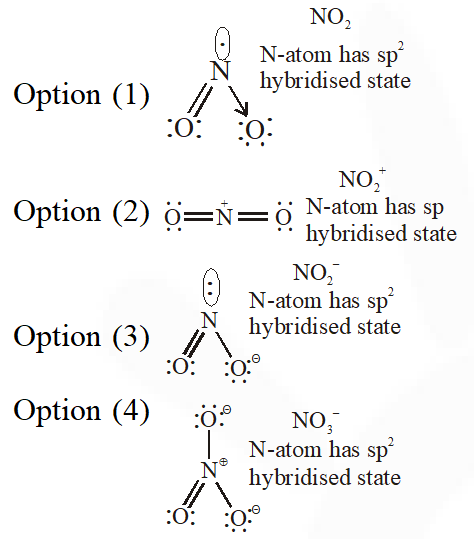Q. Which of the following species is not paramagnetic :- (1)NO (2) CO (3) $\mathrm{O}_{2}$ (4) $\mathrm{B}_{2}$ [JEE-MAINS 2017]
Ans. (2)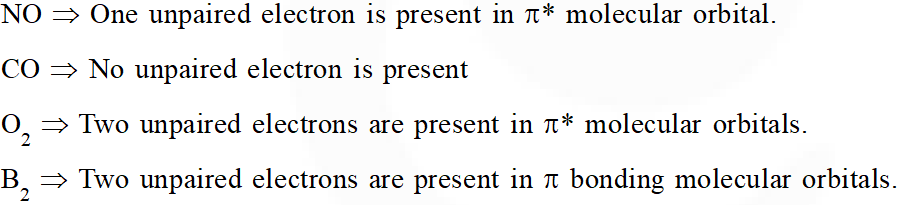Q. Total number of lone pair of electrons in $\mathrm{I}_{3}^{-} \mathrm{ion}$ is (1) 6 (2) 9 (3) 12 (4) 3 [JEE-MAINS 2018]
Ans. (2)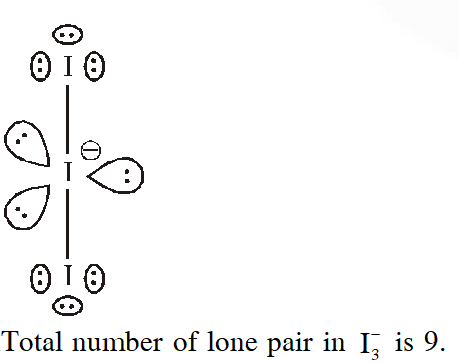Q. According to molecular orbital theory, which of the following will not be a viable molecule ? (1) $\mathrm{He}_{2}^{+}$ (2) $\mathrm{H}_{2}^{-}$ (3) $\mathrm{H}_{2}^{2-}$ (4) $\mathrm{He}_{2}^{2+}$ [JEE-MAINS 2018]
Ans. (3)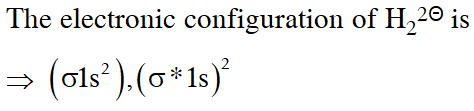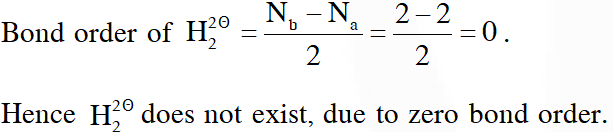Q. Which of the following compounds contain(s) no covalent bond(s) ? $\mathrm{KCl}, \mathrm{PH}_{3}, \mathrm{O}_{2}, \mathrm{B}_{2} \mathrm{H}_{6}, \mathrm{H}_{2} \mathrm{SO}_{4}$ $(1) \mathrm{KCl}, \mathrm{H}_{2} \mathrm{SO}_{4}$ (2) KC1 (3) $\mathrm{KCl}, \mathrm{B}_{2} \mathrm{H}_{6}$ (4) $\mathrm{KCl}, \mathrm{B}_{2} \mathrm{H}_{6}, \mathrm{PH}_{3}$ [JEE-MAINS 2018]
Ans. (2)

Khaby👐👐👐
Aug. 25, 2021, 9:24 p.m.
We are cet aspirant # khaby lovers 🔥🔥🔥🔥
RP
Aug. 25, 2021, 9:20 p.m.
Ghanta
HARISH
June 30, 2021, 11:48 a.m.
nice question only jee mains level
Alakh Pandey ( Physics wallah)
April 29, 2021, 6:24 p.m.
Are madharchodo randio ke aulaad gaaaaaaaaaaaaar far ke bajaaaaaaaar ke le leeeengeeee
The Real Alakh Pandey
May 31, 2023, 6:35 a.m.
Mujhe Impersonate Karta hai, बिच एस्स निग्गा
jayant joshi
April 20, 2021, 2:04 a.m.
thank you esaral for this
Karthik
Feb. 18, 2021, 5:05 p.m.
Chemical bonding is so difficult to remember structures And I want some structure
Karthik
Feb. 18, 2021, 5:04 p.m.
Chemical bonding is so difficult to remember structures
P shree mohan chandra naik
Feb. 16, 2021, 3:41 p.m.
it saved my time a lot thank you so much it really helped me a lot
Dec. 12, 2020, 3:46 p.m.
Pandya!!
Nov. 19, 2020, 9:22 a.m.
Thank you so much.....
Shsjjsj
Oct. 18, 2020, 3:23 p.m.
Proper solution with explanation needed
Hari priya
Sept. 30, 2020, 5:05 p.m.
It was really helpful... thanks a lot..😎
King happy
Sept. 26, 2020, 11:54 p.m.
Mst hai ekdum
Lohitha
Sept. 26, 2020, 7:37 p.m.
tnxs..
JEE Aspirant
Sept. 5, 2020, 12:07 a.m.
Superb work.. very helpful in revision..thanks
Manoj
Aug. 28, 2020, 10:22 a.m.
Many students will come under your course sir....thanks a lot.
Anirudh
Aug. 26, 2020, 5:09 p.m.
Good revision in this pandemic year(2020).....😃
Priya
Aug. 25, 2020, 10:52 p.m.
Too good .nyc tq so much
Chinni
Aug. 23, 2020, 11:17 p.m.
Sooper😍
harsh moon
Aug. 23, 2020, 2:14 a.m.
thank you am solving this at 2:00 am
May 31, 2023, 6:35 a.m.
Aug. 21, 2020, 2:51 p.m.
Jeep mains 2019 questions plsss DE dijiye
Rocky
Aug. 20, 2020, 6:53 p.m.
Awesome 😊
DIVYA
Aug. 14, 2020, 5:58 p.m.
Thank you so much..
Manjunathreddy
Aug. 11, 2020, 5:28 p.m.
Super work
Samarpit
July 27, 2020, 11:09 p.m.
Gr8
Israel
July 20, 2020, 2:04 p.m.
👆Awesome 👌 Thanks a lot👍
Vamsi
July 13, 2020, 9:08 a.m.
Excellent work cheers!!!!
June 23, 2020, 5:18 p.m.
Thanks a lot
Suhani
May 31, 2020, 11:41 a.m.
Thank you
Md intihaj
May 29, 2020, 3:03 p.m.
I love you esarl ......md intihaj
Anil
May 24, 2020, 10:31 a.m.
This site help me to crak mains during lockdown
Akhil
May 23, 2020, 10:17 p.m.
Nice revision, chill bro
May 20, 2020, 10 p.m.
thanks
Akshitha
May 18, 2020, 9:25 a.m.
This site is very helpful to a JEE aspirants...🙏
praveen mava
May 17, 2020, 9:39 a.m.
thank you very much
Ethane
May 9, 2020, 12:52 p.m.
Gooooooooooooooooooooooooooooooooooooooooooooooooooooooooooopoooooood
Propane
May 31, 2023, 10:11 a.m.
बिच एस्स निग्गा
Yashveer singh
April 22, 2020, 1:44 a.m.
thankyou very much
hari
April 20, 2020, 7:28 p.m.
super revision
Koushika
April 4, 2020, 10:18 p.m.
Thank you so much